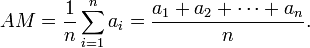# Measures of central tendency

### Last updated: 22 OCT 2023

Measures of central tendency (averages) - a single value that attempts to describe a set of data by identifying "the central position" within that set of data. Central position can be defined in many ways therefore we have many averages.

The three most common measures of central tendency are the mean, the median, and the mode.

## Arithmetic mean

Arithmetic mean - the sum of the numbers divided by the number of numbers in a set of data. This is one of the most intuitive ways of population evaluation.a - numbers for which the average is calculated, n - number of numbers in a set of data

Example

Let's take group B where we have people with the following age distribution : 36, 36, 37, 38, 39, 40, 41, 42, 44, 47. To calculate the arithmetic mean we need to sum up all elements in the group and then divide them by the number of these elements. The arithmetic mean (average) for this example is equal 400 / 10 = 40.

## Median

Median - the numerical value separating the higher half of a sample, a population, or a probability distribution, from the lower half. The median is also second quartile, fifth decile, ...

Example

Let's take again our group B : 36, 36, 37, 38, 39, 40, 41, 42, 44, 47. To find the median we need to order our sample and find the middle value. As we have 10 elements in the sample the middle value will be between 5th and 6th element. In group B median is equal to 39,5.

## Mode

Mode is the number that appears most often in a set of numbers.

Example

To find the mode we need to find a value that appears most often in the sample. In the group B : 36, 36, 37, 38, 39, 40, 41, 42, 44, 47 mode is equal 36.

Other averages that you will be able to find are : weighted mean, geometric mean, or harmonic mean.